# Common Core: High School - Geometry : Construct Tangent Lines from Outside a Circle: CCSS.Math.Content.HSG-C.A.4

## Example Questions

← Previous 1

### Example Question #1 : Construct Tangent Lines From Outside A Circle: Ccss.Math.Content.Hsg C.A.4

Calculate a point that is tangent to the circle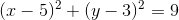and passes through the origin.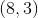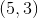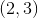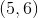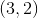Explanation:

explain

### Example Question #1 : Construct Tangent Lines From Outside A Circle: Ccss.Math.Content.Hsg C.A.4

Calculate a point that is tangent to the circleand passes through the origin.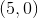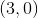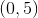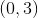Explanation:

To construct a line that is tangent to a point on the circle and passes through the origin, recall what it means for a line to be "tangent". A line that is tangent to a point on a circle means that the line will only touch the circle at that specific point.

Given the equation of the circle,the center and radius of the circle can be determined.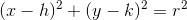The center is located at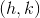and the radius is.

Therefore, the center is located atand the radius is three. Plotting the circle and tangent line to the origin results in the following.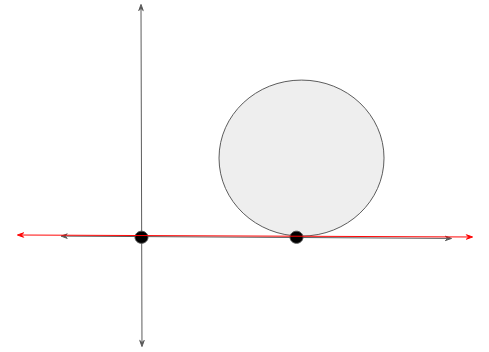### Example Question #2 : Construct Tangent Lines From Outside A Circle: Ccss.Math.Content.Hsg C.A.4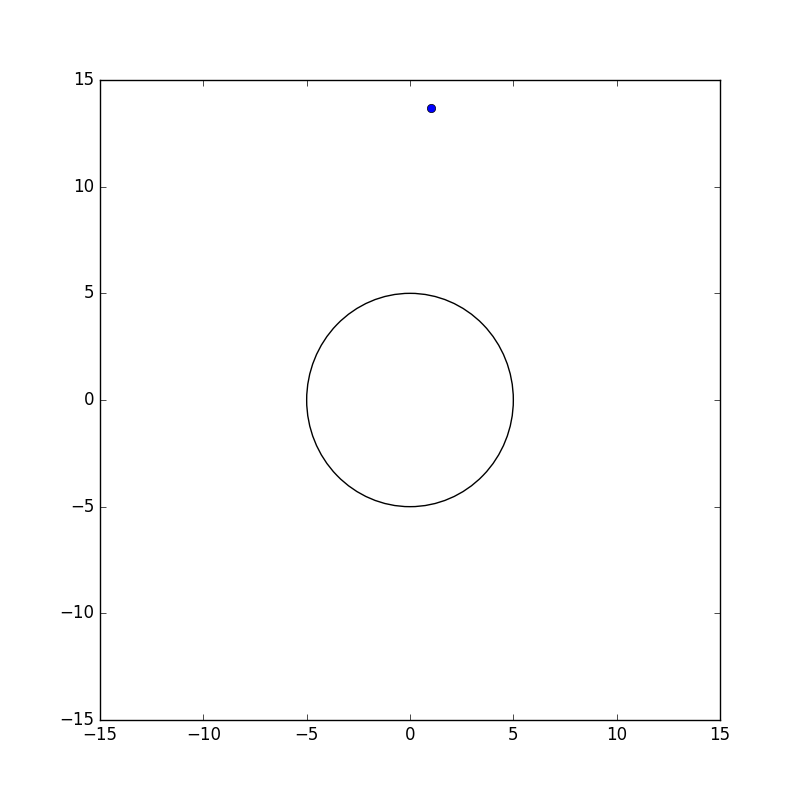Construct a line that is tangent to a point on the circle and passes through the point plotted outside the circle.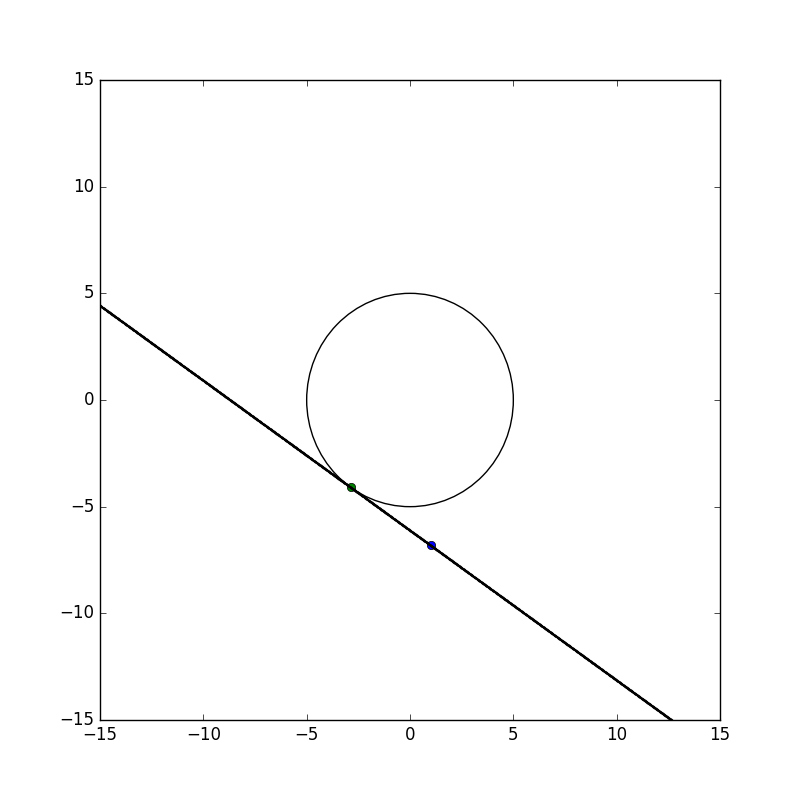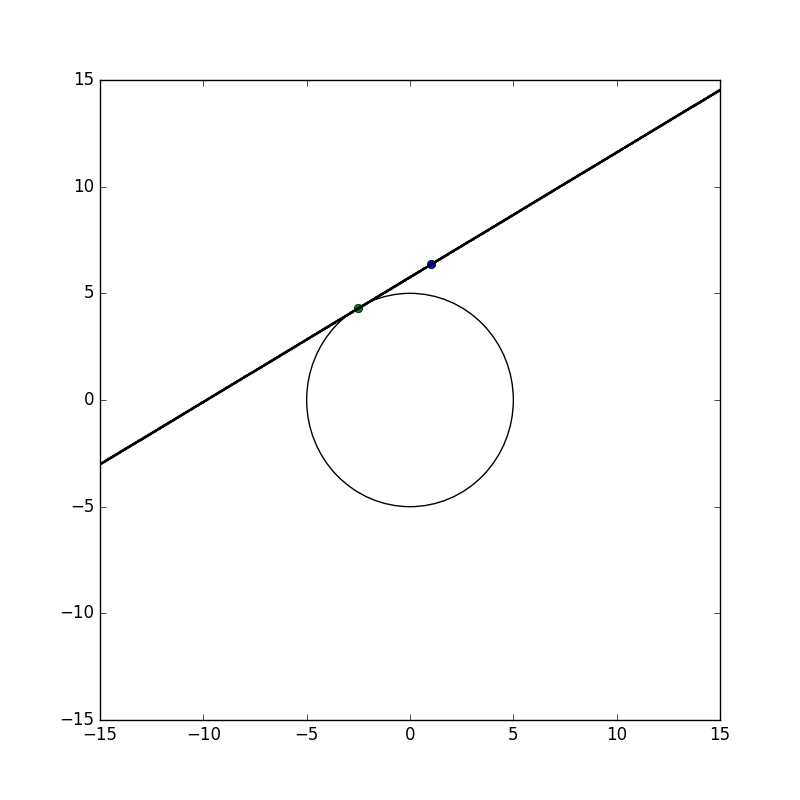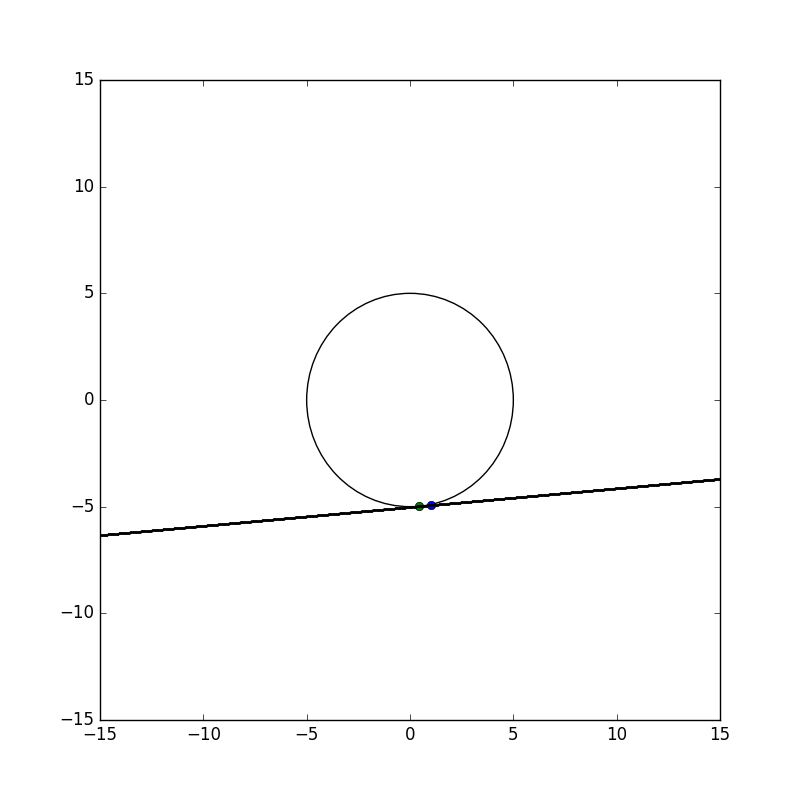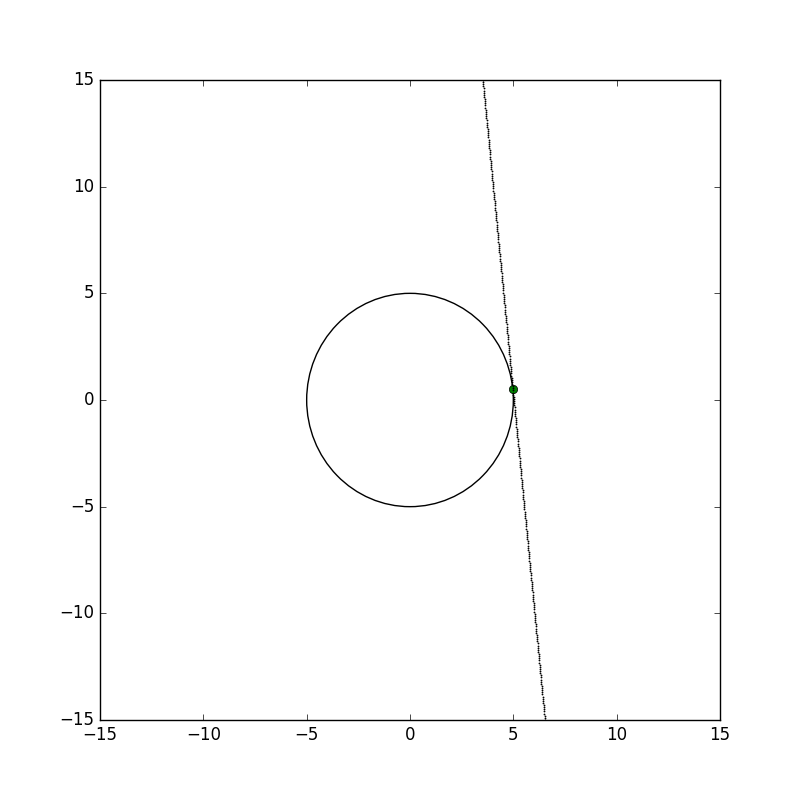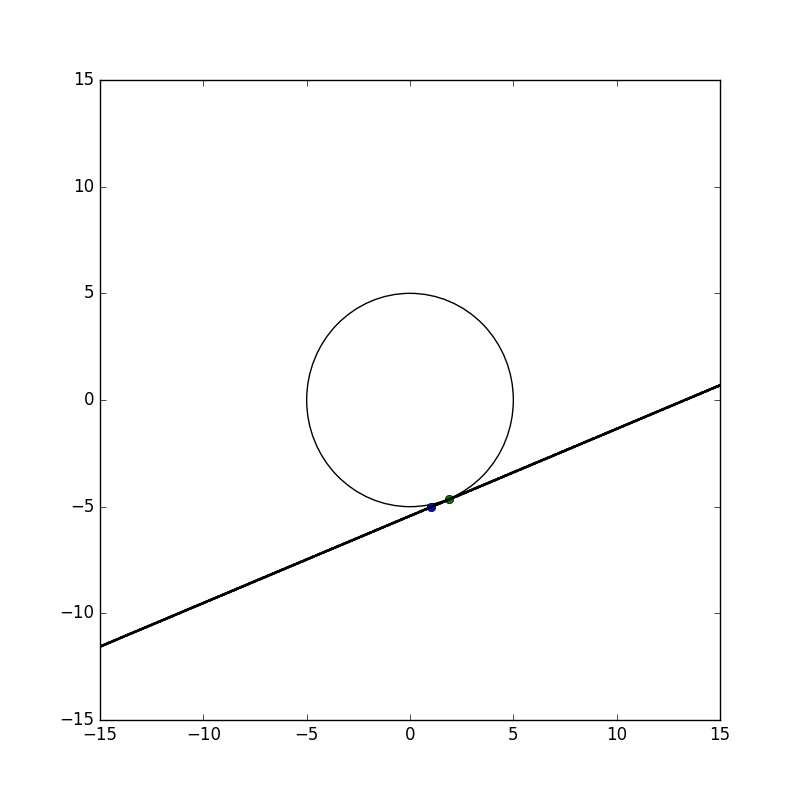Explanation:

To construct a line that is tangent to a point on the circle and passes through the point outside the circle, recall what it means for a line to be "tangent". A line that is tangent to a point on a circle means that the line will only touch the circle at that specific point.

Using the plotted circle and the given point, two potential lines can be drawn that will touch the circle at one point. One possible line would touch the circle on the left half of the circumference while the other potential line would touch the circle on the right half.Constructing a potential tangent line, a point can be plotted on the circle as follows.

From here, connect the given point outside the circle to the point on the circle with a straight line. Thus resulting in a tangent line to the circle.### Example Question #1 : Construct Tangent Lines From Outside A Circle: Ccss.Math.Content.Hsg C.A.4

Determine whether the statement is true or false.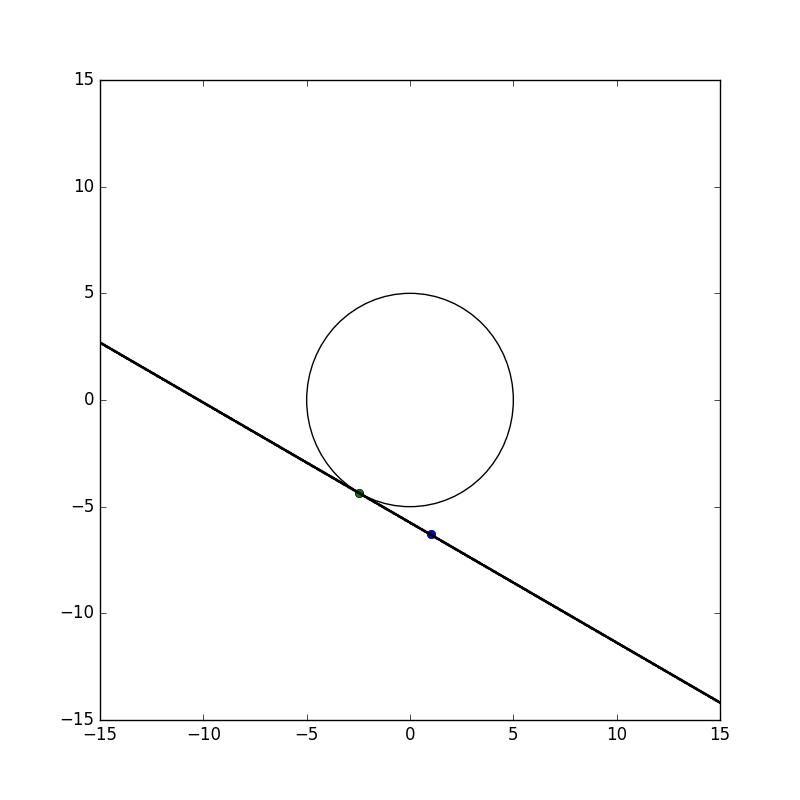The line is tangent to the circle.

True

False

True

Explanation:

To construct a line that is tangent to a point on the circle, recall what it means for a line to be "tangent". A line that is tangent to a point on a circle means that the line will only touch the circle at that specific point.

Looking at the image, it is seen that the line only touches the circle once therefore, the line is tangent to the circle. Thus this statement is true.### Example Question #4 : Construct Tangent Lines From Outside A Circle: Ccss.Math.Content.Hsg C.A.4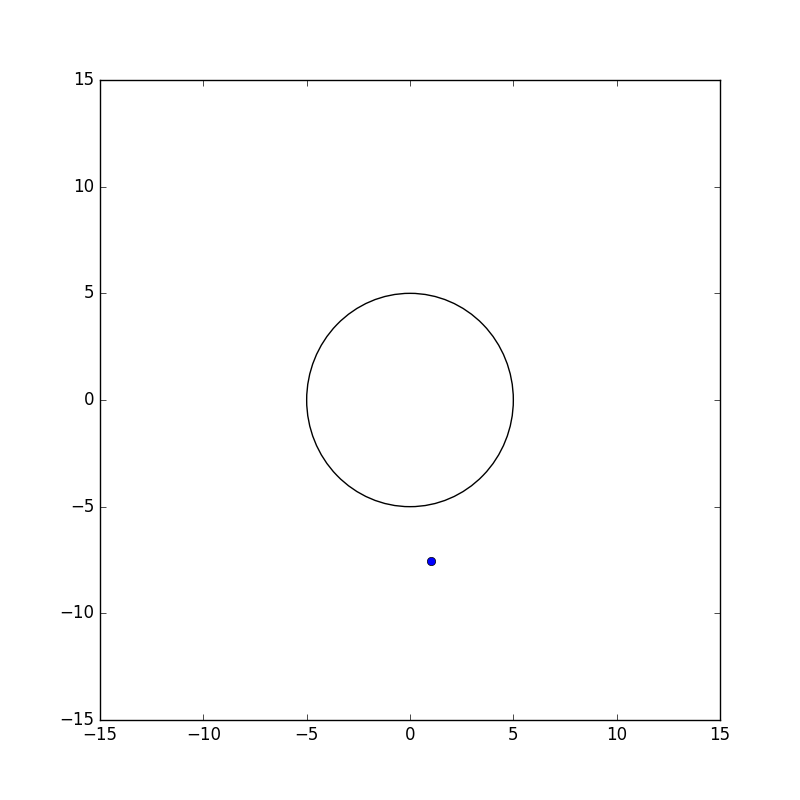Construct a line that is tangent to a point on the circle and passes through the point plotted outside the circle.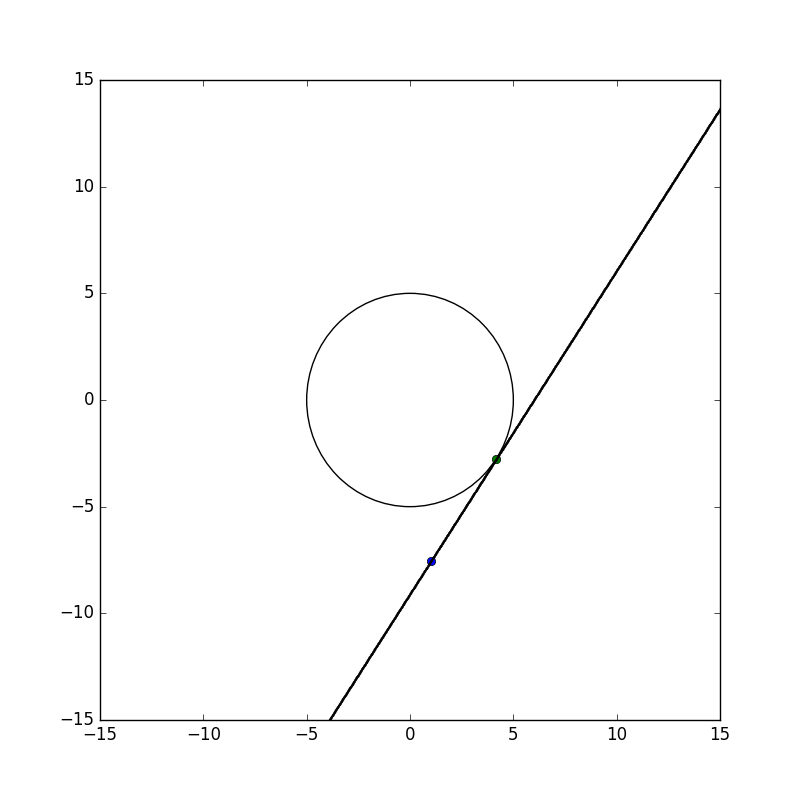Explanation:

To construct a line that is tangent to a point on the circle and passes through the point outside the circle, recall what it means for a line to be "tangent". A line that is tangent to a point on a circle means that the line will only touch the circle at that specific point.

Using the plotted circle and the given point, two potential lines can be drawn that will touch the circle at one point. One possible line would touch the circle on the left half of the circumference while the other potential line would touch the circle on the right half.Constructing a potential tangent line, a point can be plotted on the circle as follows.

From here, connect the given point outside the circle to the point on the circle with a straight line. Thus resulting in a tangent line to the circle.### Example Question #2 : Construct Tangent Lines From Outside A Circle: Ccss.Math.Content.Hsg C.A.4

Determine whether the statement is true or false.

Given a circle, a tangent line to the circle can be constructed if it intersects the circle at two points.

False

True

False

Explanation:

To construct a line that is tangent to a point on the circle, recall what it means for a line to be "tangent". A line that is tangent to a point on a circle means that the line will only touch the circle at that specific point.

Therefore, by definition the statement is false.

### Example Question #2 : Construct Tangent Lines From Outside A Circle: Ccss.Math.Content.Hsg C.A.4

Determine whether the statement is true or false.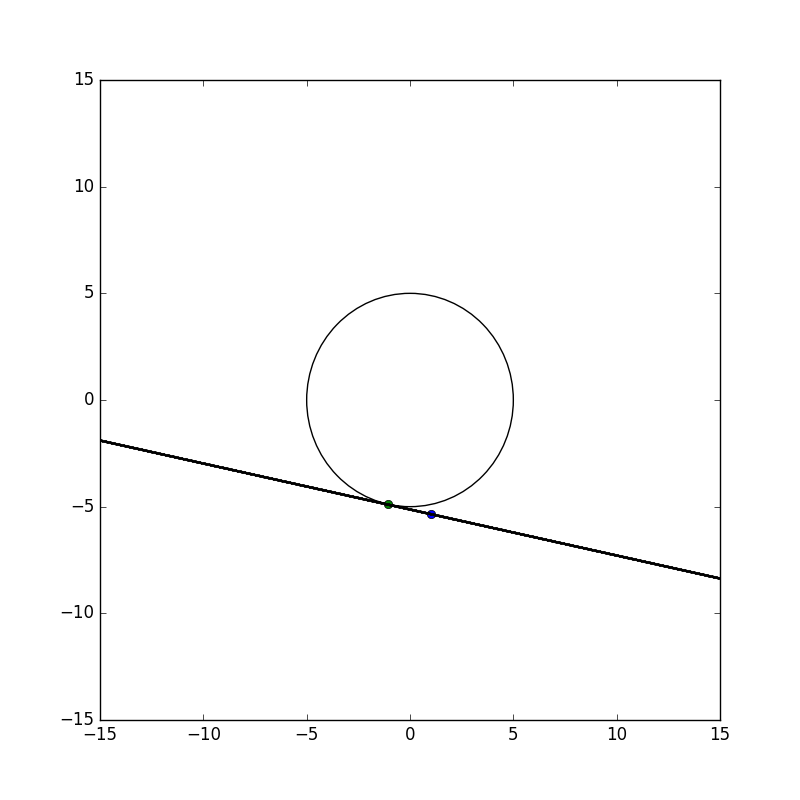The line is tangent to the circle.

True

False

True

Explanation:

To construct a line that is tangent to a point on the circle, recall what it means for a line to be "tangent". A line that is tangent to a point on a circle means that the line will only touch the circle at that specific point.

Therefore, looking at the graphit is seen that the line only intersects the circle once. Thus, the statement "The line is tangent to the circle." is true.

### Example Question #7 : Construct Tangent Lines From Outside A Circle: Ccss.Math.Content.Hsg C.A.4

Calculate a point that is tangent to the circleand passes through the point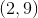.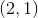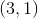Explanation:

To construct a line that is tangent to a point on the circle and passes through the point, recall what it means for a line to be "tangent". A line that is tangent to a point on a circle means that the line will only touch the circle at that specific point.

Given the equation of the circle,the center and radius of the circle can be determined.The center is located atand the radius is.

Therefore, the center is located atand the radius is three. Plotting the circle and tangent line to the pointresults in the following.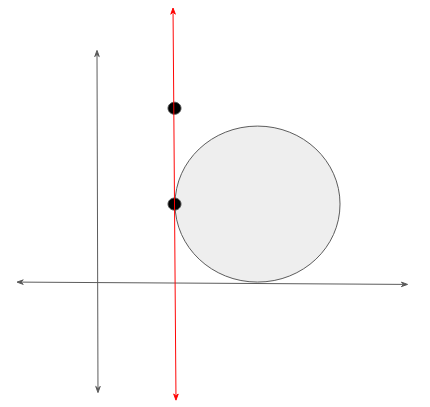Therefore, the point on the circle that creates a tangent line,.

### Example Question #8 : Construct Tangent Lines From Outside A Circle: Ccss.Math.Content.Hsg C.A.4

Calculate a point that is tangent to the circleand passes through the point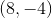.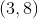Explanation:

To construct a line that is tangent to a point on the circle and passes through the point, recall what it means for a line to be "tangent". A line that is tangent to a point on a circle means that the line will only touch the circle at that specific point.

Given the equation of the circle,the center and radius of the circle can be determined.The center is located atand the radius is.

Therefore, the center is located atand the radius is three. Plotting the circle and tangent line to the pointresults in the following.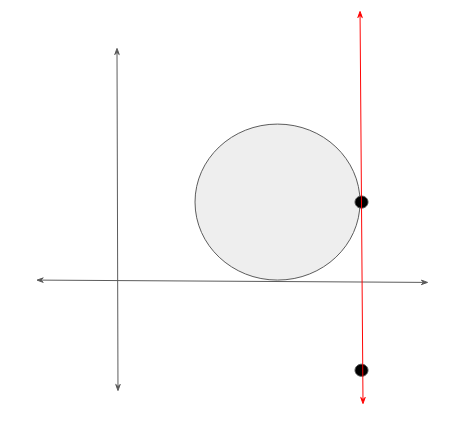Therefore, the point on the circle that creates a tangent line with the point given is.

### Example Question #3 : Construct Tangent Lines From Outside A Circle: Ccss.Math.Content.Hsg C.A.4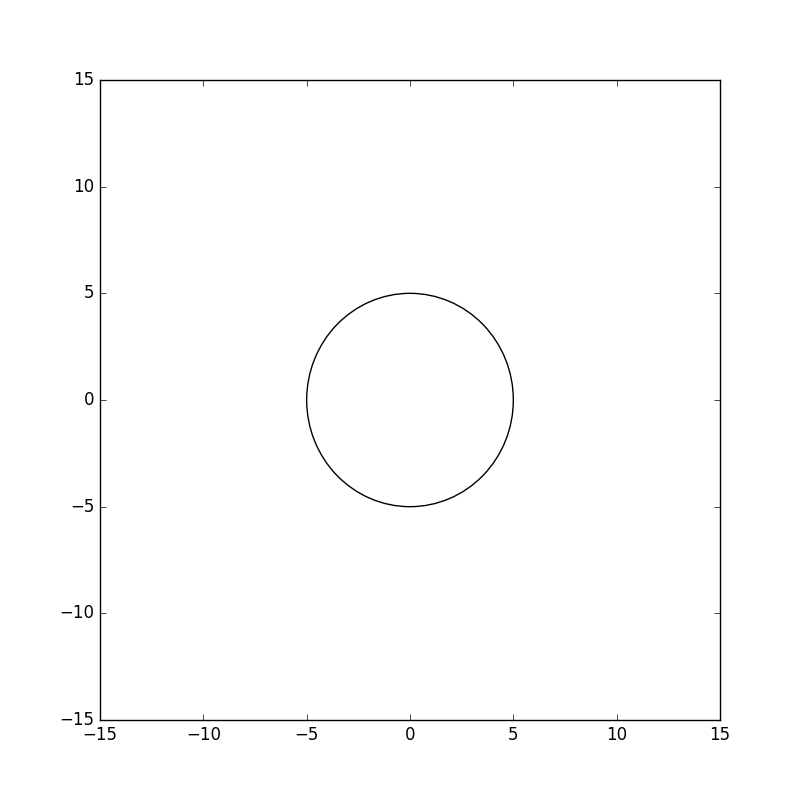Construct a line that is tangent to the circle.None of the images depict a tangent line.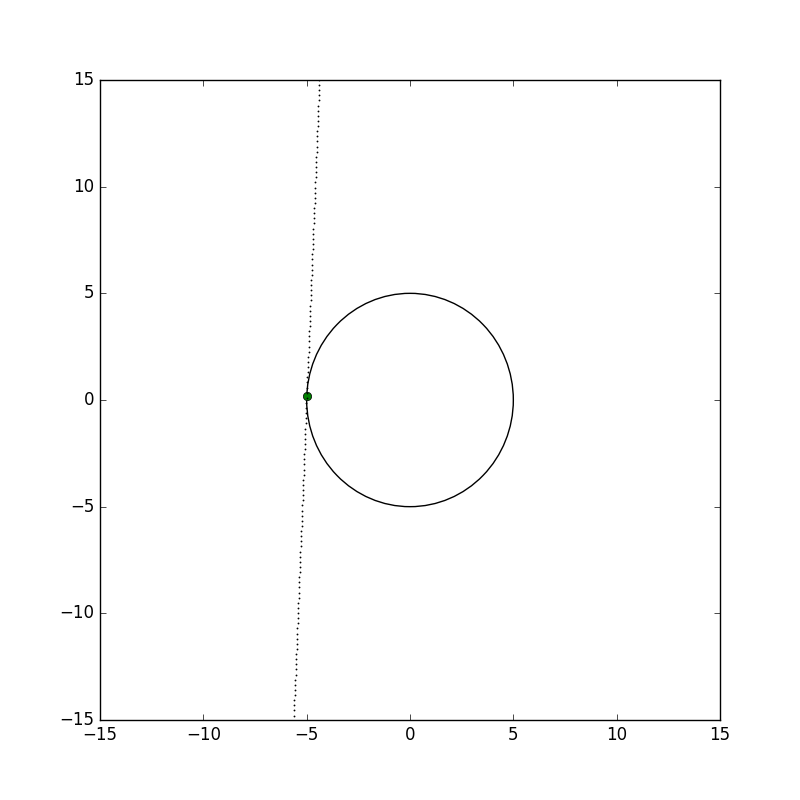Explanation:

To construct a line that is tangent to a point on the circle, recall what it means for a line to be "tangent". A line that is tangent to a point on a circle means that the line will only touch the circle at that specific point.

Using the plotted circle one possible line would touch the circle is as follows.Thus resulting in a tangent line to the circle.

← Previous 1

### All Common Core: High School - Geometry Resources